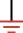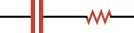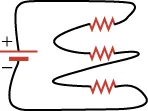Physics

# Multiple Choice

PhysicsMultiple Choice

### Multiple Choice

#### 19.1Ohm's law

30 .
What are the SI units for electric current?
1. $\text{C/s}$
2. $\text{e/s}$
3. $-\text{e}/\text{s}$
4. $\text{C/s}^2$
31 .
What quantity is used to describe the ability of a material to limit current flow?
1. C/m
2. C/s
3. Ω
4. V
32.

The equivalent unit for an ohm is a ________.

1. V/A
2. C/m
3. $A V A V$
4. V/s
33 .
You put $9.0\,\text{V}$ DC across resistor $R_1$ and measure the current through it. With the same voltage across resistor $R_2$, you measure twice the current. What is the ratio $\frac{R_1}{R_2}$?
1. 1
2. $\frac{1}{2}$
3. 4
4. 2

#### 19.2Series Circuits

34.

What does the circuit element shown represent?1. a battery
2. a capacitor
3. the ground
4. a switch
35.

How many 10-Ω resistors must be connected in series to make an equivalent resistance of 80 Ω?

1. 80
2. 8
3. 20
4. 40
36.

Which two circuit elements are represented in the circuit diagram?1. a battery connected in series with an inductor
2. a capacitor connected in series with a resistor
3. a resistor connected in series with a battery
4. an inductor connected in series with a resistor
37.

How much current will flow through a 10-V battery with a 100-Ω resistor connected across its terminals?

1. 0.1 A
2. 1.0 A
3. 0
4. 1,000 A

#### 19.3Parallel Circuits

38.

A 10-Ω resistor is connected in parallel to another resistor R . The equivalent resistance of the pair is 8 Ω. What is the resistance R?

1. 10 Ω
2. 20 Ω
3. 30 Ω
4. 40 Ω
39.

Are the resistors shown connected in parallel or in series? Explain.1. The resistors are connected in parallel because the same current flows through all three resistors.
2. The resistors are connected in parallel because different current flows through all three resistors.
3. The resistors are connected in series because the same current flows through all three resistors.
4. The resistors are connected in series because different current flows through all three resistors.

#### 19.4Electric Power

40 .
Which equation below for electric power is incorrect?
1. $P = I^2 R$
2. $P = \frac{V}{R^2}$
3. $P = IV$
4. $P = \frac{V^{2}}{R}$
41 .
What power is dissipated in a circuit through which $0.12\,\text{A}$ flows across a potential drop of $3.0\,\text{V}$?
1. $0.36\,\text{W}$
2. $0.011\,\text{W}$
3. Voltage drop across is $5\,\text{V}$.
4. $2.5\,\text{W}$
42 .
How does a resistor dissipate power?
1. A resistor dissipates power in the form of heat.
2. A resistor dissipates power in the form of sound.
3. A resistor dissipates power in the form of light.
4. A resistor dissipates power in the form of charge.
43.

What power is dissipated in a circuit through which 0.12 A flows across a potential drop of 3.0 V?

1. 0.36 W
2. 0.011 W
3. 5 V
4. 2.5 W
Order a print copy

As an Amazon Associate we earn from qualifying purchases.